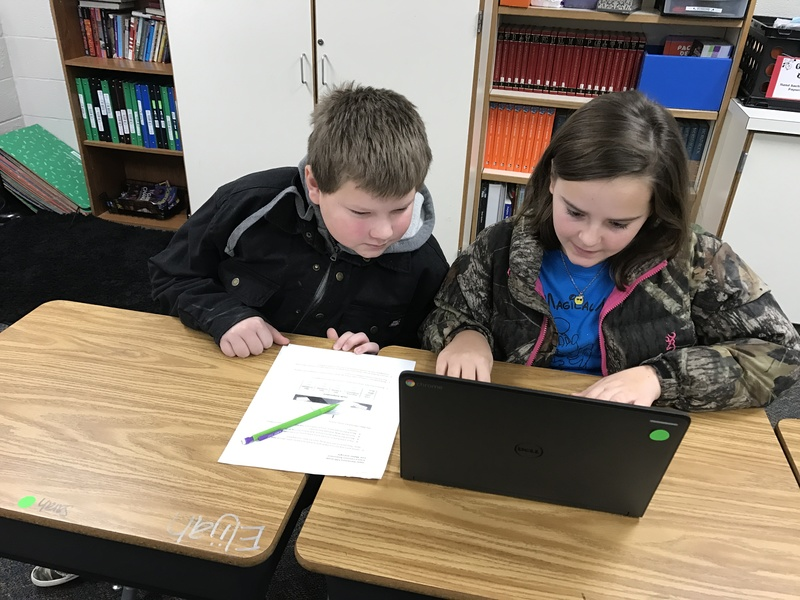EDIT MAIN## Math

Fifth grade mathematics is about:

• developing fluency with addition and subtraction of fractions, and developing understanding of the multiplication of fractions and of division of fractions in limited cases
• extending division to two-digit divisors, integrating decimal fractions into the place value system and developing understanding of operations with decimals to hundredths, and developing fluency with whole number and decimal operations
• developing understanding of volume